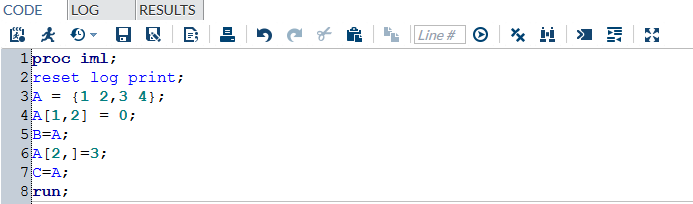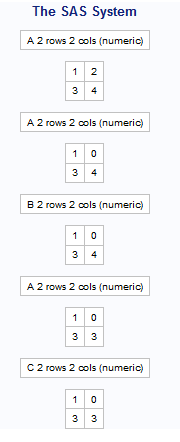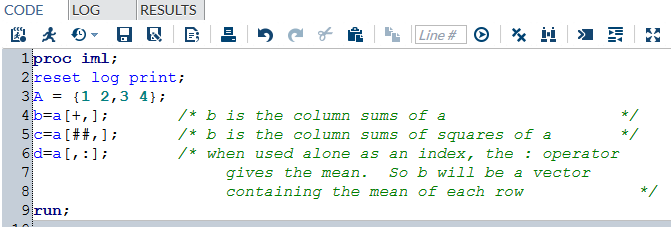Tavish Srivastava — Published On November 4, 2014 and Last Modified On August 27th, 2021

In the last article on IML (here) , we introduced you to the world of Matrix language on SAS. We also talked about some basic commands on IML. This article will take you a step further in the same world. After reading this article, you can take up problem solving using SAS IML. In some of the coming articles on IML, we will also take some examples of cases where IML will be very handy.

### A Quick Recap

Following code will help you recall all the commands we discussed in the last article. Try guessing the output of the commands before looking at the output below.

proc iml;

reset log print;

A = {1 2,3 4};

B=t(A);

C= inv(A);

D = A*C;

run;Following are the description of each matrix defined in this code :

A : Original Matrix

B : Transpose of Matrix A

C : Inverse of Matrix A

D : Matrix multiplication of A and B which should ideally be an identity matrix (Using property of Inverse).

Now let’s add a few more types of command on IML in our dictionary.

### Replacing elements of a Matrix

In many cases we need to manipulate individual datapoint to fix outliers or missing values. To do this operation in IML, you can use the following method (B has one value replaced while C has an entire column replaced) :

proc iml;

reset log print;

A = {1 2,3 4};

A[1,2] = 0;

B=A;

A[2, ] = 3;

C = A;

run;

###Summarize operators

Operation like normalization of probabilities, needs you to collapse one dimension of the matrix.  Following code can be used to collapse row or column :

proc iml;

reset log print;

A = {1 2,3 4};

c = a[+,];

c=a[##,];

d=a[,:];

run;### Control statements

For making any logic, we always need control statements/conditional statements. IML supports all IF-THEN, DO-WHILE, DO-UNTIL statements. Following are a commands which will help you write conditional statements :

proc iml;

reset log print;

A = {1 2,3 4};

B= {2 3,4 5};

if A < B then

msg = “all (A<B)”;

else msg = “some elements of A is greater than or equal to the corresponding element of B”;

run;

Note that in IML, the condition is applied on every element. If element wise operation at all row and column gives a TRUE output, the final output is TRUE. Following is an example of a do-end statement :

proc iml;

x=1:5;

sum1=0;

do i = 1 to ncol(x);

sum1=sum1+x[i];

end;

sum2 = sum(x);

print sum1 sum2;

run;

### Concatenation Operators

Very often we try merging or appending the datasets. IML provides very easy way to do these operations. Following commands can be used for merging (horizontal concatenate) and appending (vertical concatenate) :

proc iml;

a = {1,2,3,4,5};

b={3,5,4,1,3};

c={0,1,0,0,1};

x=a||b||c;

y=a//b;

print x;

print y;

run;

### Logical Operators

Multiple conditions can be used together to form complex conditions on IML. Following is a simple code which demonstrates the same :

proc iml;

do x=-3 to 3;

if (x>=-2) & (x<=2) then

y = sqrt(4-x##2);

else

y=”Unable to compute the function. “;

print y;

end;

run;

### End Notes

This command list was not exhaustive but will definitely enable you to fulfill most of the purposes. In our following articles we will pick up some iterative problems like formulation of Newton-Raphson, Solving Linear Regression, Linear Programming etc. to demonstrate the usage of IML programming.

Did you find the article useful? Have you used IML before?  Do you plan to use IML in any of your business problems? If yes, share with us how IML programming will simplify or enable your analysis.

### If you like what you just read & want to continue your analytics learning, subscribe to our emails, follow us on twitter or like our facebook page.